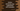# Python dictionary pop method explanation with examples## Python dictionary pop method explanation with examples:

In this post, we will learn how to use the pop method of Python dictionary with different examples. We will learn the definition of the pop method and how to use it.

### Definition of pop:

The pop method is used to remove an element from a dictionary. We have to pass the key to this method and if that key is found, it removes that key and returns the value.

The pop method is defined as like below:

``dict.pop(k[, v])``

Where,

• k is the key to search for removal in the dictionary.
• v is an optional value. If the key is not found in the dictionary, this method will return this value.

#### Return value:

• This method returns the value of the provided key.
• If the key is not found in the dictionary, it returns the default value i.e. v if it is provided.
• If the key is not found in the dictionary and if the default value v is also not provided, it throws KeyError exception.

Let’s learn how it works with different examples.

### 1. Example of python dictionary pop:

Let’s take a look at the below example program:

``````given_dict = {'one': 1, 'two': 2, 'four': 4}

print(f'Dictionary: {given_dict}')

removed_value = given_dict.pop('four')

print(f'Element with value {removed_value} is removed')
print(f'Dictionary: {given_dict}')``````

Here,

• given_dict is the given dictionary.
• We are using pop to remove an item from the dictionary with key ‘four’. The returned value of pop is saved in removed_value variable.
• Before pop is called, it prints the dictionary.
• The last two lines are printing the removed value, i.e. the return value of pop and the dictionary after the pair is removed.

If you run this program, it will print the below result:

``````Dictionary: {'one': 1, 'two': 2, 'four': 4}
Element with value 4 is removed
Dictionary: {'one': 1, 'two': 2}``````

As you can see here, the pair with ‘four’ as the key is removed from the dictionary.

### 2. Example of exception:

As I explained above, it throws a KeyError exception if the key is not found in the dictionary.

For example:

``````given_dict = {'one': 1, 'two': 2, 'four': 4}

print(f'Dictionary: {given_dict}')

removed_value = given_dict.pop('three')

print(f'Element with value {removed_value} is removed')
print(f'Dictionary: {given_dict}')``````

In this example, we are passing ‘three’ to the pop method. But, this key is not there in the dictionary. So, it will throw a KeyError.

``````Traceback (most recent call last):
File "example.py", line 5, in <module>
removed_value = given_dict.pop('three')
KeyError: 'three'``````

We can use a try-catch block to avoid this.

``````given_dict = {'one': 1, 'two': 2, 'four': 4}

print(f'Dictionary: {given_dict}')

try:
removed_value = given_dict.pop('three')

print(f'Element with value {removed_value} is removed')
print(f'Dictionary: {given_dict}')
except KeyError:

It will not throw any exception and print the line in the KeyError block.

``````Dictionary: {'one': 1, 'two': 2, 'four': 4}

### 3. Example with default value:

Instead of using a try-except block, we can also pass the default value as the second parameter to pop. It will not throw any exception and return that value if the key is not there in the dictionary.

For example,

``````given_dict = {'one': 1, 'two': 2, 'four': 4}

print(f'Dictionary: {given_dict}')

removed_value = given_dict.pop('three', -1)

if removed_value == -1:
else:
print(f'Element with value {removed_value} is removed')
print(f'Dictionary: {given_dict}')``````

In this example, we are passing -1 as the default value. Since ‘three’ is not in the dictionary, it will return the default value, i.e. -1.

The if-else block checks if the returned value is equal to the default value or not. If yes, it prints that the key is not found in the dictionary.

Make sure that there is no value in the dictionary equal to the default value.

So, if you run this program, it will print:

``````Dictionary: {'one': 1, 'two': 2, 'four': 4}